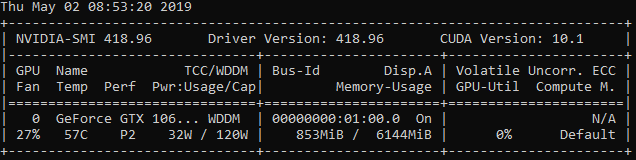# GPU not being used

I’m trying to run my CNN training and testing on GPU but it’s not using GPUI am stating

`model = models.CNN1().cuda()`
`inputs = Variable(inputs).cuda()`
`labels = Variable(labels).cuda()`
`outputs = model(inputs)`

And when I print out device, it does say `Device: cuda:0`

Please help.It looks like the GPU0 is being used.
What does `nvidia-smi` show? Any memory allocation and utilization?Do you see the utilization and memory increase at some point or is it the whole time at 0%?
Also, what kind of model are you using? Is it quite small so that the workload might be minimal compared to the data loading, processing, etc.?

It does change to 3%, 5% sometimes, then back to 0%.
I have various cnn models (none producing great results yet). Shallow and deep, none use GPU like I want it to.

example shallow model:

``````class CNN1(nn.Module):
def __init__(self):
super(CNN1, self).__init__()
self.layer1 = nn.Sequential(nn.Conv2d(1, 10, 5, 1),
nn.ReLU(),
nn.MaxPool2d(2, 2))
self.fc1 = nn.Linear(170, 2)

def forward(self, x):
x = x.unsqueeze(1)
x = self.layer1(x)
x = x.reshape(x.size(0), -1)
x = self.fc1(x)
return x
``````

example deeper model:

``````class CNN2(nn.Module):
def __init__(self):
super(CNN2, self).__init__()
self.layer1 = nn.Sequential(
nn.ReLU(),
nn.MaxPool2d(kernel_size=2, stride=2))
self.layer2 = nn.Sequential(
nn.ReLU(),
nn.MaxPool2d(kernel_size=2, stride=2))
self.drop_out = nn.Dropout(0.5)
self.fc1 = nn.Linear(576, 1000)
self.fc2 = nn.Linear(1000, 1000)
self.fc3 = nn.Linear(1000, 100)
self.fc4 = nn.Linear(100, 2)

def forward(self, x):
x = x.unsqueeze(1)
out = self.layer1(x)
out = self.layer2(out)
out = out.reshape(out.size(0), -1)
out = out.float()
out = self.drop_out(out)
out = self.fc1(out)
out = self.fc2(out)
out = self.fc3(out)
out = self.fc4(out)
return out
``````

The CNN2 model sometimes shows 14%, 24%, then 0% mostly. But Task Manager performance still shows 0% for GPULoading the data in the `Dataset`'s `__getitem__` is a good idea.
Are you using multiple workers in your `DataLoader` (`num_workers > 0`)?
Is your data stored on a local SSD or are you pulling it from a network device?

PS: I would recommend to use `tensor.view` instead of `tensor.reshape` in your `forward` method.ThanksI tried using `num_workers > 0` but no difference. I even set batch size to 1024. CPU shows 100% and GPU 0%.
My data is stored locally.

Are you applying a lot of preprocessing?
If you are using `PIL`, you might use `Pillow-SIMD`, which is a drop-in replacement for Pillow with SIMD.
Could you post the `__getitem__` method so that we can have a look at potentially speedups?

``````    def __getitem__(self, index):
with open(self.folder + str(index) + '.txt', 'r') as file:
buffer = buffer.split('\n')
label = int(buffer.pop(0).split('\t'))
buffer.pop()
image = []
for line in buffer:
line = line.split('\t')[1:-1]
line = [float(x) for x in line]
image.append(line)
``````

Oh, your images are stored in text files?
If that’s the case, I could suggest to load them all once and store them in an image format or binary format using `torch.save`.
This should speed thing up.

Yes I saved them in text files. Initially I saved them as pickle objects in one file and loaded all in a list in the `__init__()` but that filled up my RAM and all crashed, so I then saved the images in separate text files.

I’m not sure how you want me to use torch.save for saving image files. I thought that was for saving the model? Sorry, please could you explain? Thanks.

`torch.save` can also save tensors, not only the `state_dict`.
You could add the following line to your current `__getitem__` and store each image tensor in a folder corresponding to the label:

``````...
for line in buffer:
...
x = torch.tensor(image)
torch.save(x, self.folder + '/' + str(label) + '/' + str(index) + '.pth')
return x, torch.tensor(label)
``````

This will save each image tensor in a folder given by `str(label)`.
The file names won’t be ascending, i.e. you might end up with:

``````root/
── 0/
│   ├── 24.pth
│   ├── 4678.pth
│   ├── 4679.pth
│   └── ...
├── 1/
│   ├── 0.pth
│   └── 1.pth
``````

After saving all files (just run this code for one single epoch), you should rewrite your `Dataset` by providing the file paths to its `__init__` and just loading each image tensor using `torch.load` in `__getitem__`.
Let me know if you get stuck somewhere.

PS: Alternatively, you could also save the images using `PIL` and later just use an `ImageFolder` as your dataset.

I created the files using torch.save and fixed my Dataset code. But still getting 0% GPU usagePlease help.

Okay I tried that and found that time is not decreasing, or is it?

``````Epoch: [0/3]         Time  2.614 ( 2.614)
Epoch: [0/3]	        Time  0.595 ( 0.595)
Epoch: [0/3]	        Time  0.592 ( 0.592)
...
Epoch: [0/3]        Time  0.586 ( 0.586)
Epoch: [0/3]        Time  0.596 ( 0.596)
``````

What do you think I should do?What kind of drive are you using? Is The data on a SSD or HDD?

My data is on HDD